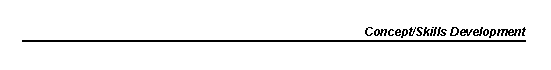1. "Law of Conservation of Mass does not apply to atoms."

Students may be able to repeat the Law of Conservation of Mass, but see no problem with atoms disappearing or appearing to balance equations.

2. "Elements can form other elements."

Several students when questioned about the appearance of copper on magnesium ribbon in copper sulfate remarked that the brown solid came from the magnesium. They thought it was somehow present in the magnesium even though they agreed that magnesium was an element.

3. "All solutions are pure liquids."

Most students, early in the year, do not distinguish between a solution and a pure liquid. Teachers and textbooks perpetuate this notion by labelling solutions as 0.1 M CuSO 4 before students understand anything about molarity. It would probably be better to label such a solution as "CuSO 4 solution" and to demonstrate for students how such solutions are prepared. One could also demonstrate that when a pure liquid is evaporated there is no residue, but when a solution is evaporated there is often a residue.

4. "There is such a thing as an unbalanced chemical equation."

An equation, by definition, is an expression in which the components on either side of the equality sign are identical. For example, in the equation 4x = 32, we are told that multiplication of x by 4 yields the quantity 32. It follows that x must be 8, and that the equation can be rewritten as 4 x 8 = 32.

A chemical equation is not a mathematical equation in the strict sense. The left side of the equation represents the reactants and the right side the products. They are joined by an arrow or "yields" sign that, for all intents and purposes, may be taken as an equality sign. The chemical equation is an expression of the Law of Conservation of Matter. Equal numbers of atomic representations must appear on both sides of the arrow. In the expressions given below, one is a chemical equation and the other is a chemical statement:

a. CaCO 3 (s) + HCl(aq) ---> CaCl 2 (aq) + CO 2 (g) + H 2 O(l)

b. CaCO 3 (s) + 2HCl(aq)---> CaCl 2 (aq) + CO 2 (g) + H 2 O(l)

In expression (a), the number of chlorine atoms and hydrogen atoms are unequal on both sides of the arrow. Placing a 2 in front of the HCl in the expression achieves the desired (and required) equality. Hence, expression (b) fulfills the definition of an equation, but expression (a) does not. Expression (b) is often called a "balanced chemical equation," and expression (a) is called an "unbalanced chemical equation." Strictly speaking, expression (a) is not an equation at all since the equality requirement is not fulfilled, and expression (b) is an equation and therefore does not need the adjective "balanced" since it is a redundancy, by definition. Common usage allows us to call both expressions equations, but according to our definition, there is no such thing as an unbalanced equation.